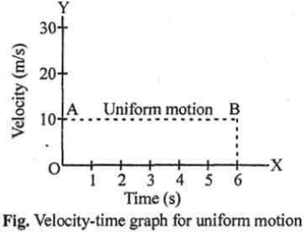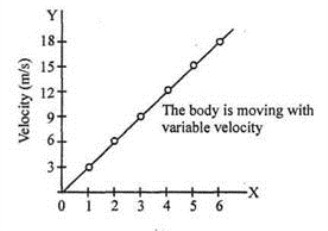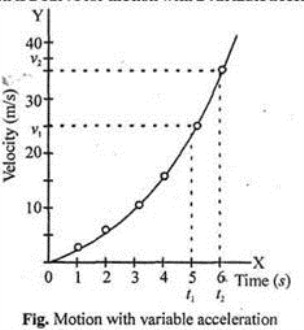# CBSE 9th Physics | Motion: Concepts

## REST

An object is said to be at rest if it does not change its position with respect to its surroundings with the passage of time.

## MOTION

A body is said to be in motion if its position changes continuously with respect to the surroundings (or with respect to an observer) with the passage of time.

We know that earth is rotating about its axis and revolving around the sun. The stationary objects like your class-room, a tree and the lamp posts etc. do not change their position with respect to each other i.e. they are at rest. Although earth is in motion.

To an observer situated outside the earth say in a space ship, your classroom, trees etc. would appear to be in motion. Therefore, all motions are relative.

There is nothing like absolute motion. If you move with book in your hand, book is not moving with respect to you.

## DISTANCE

The distance travelled by a body is the actual length of the path covered by a moving body irrespective of the direction in which the body moves.

## DISPLACEMENT

When a body moves from one position to another, the shortest (straight line) distance between the initial position and final position of the body, along with direction, is known as its displacement.

The S.I. unit of displacement is meter (m). Displacement is a vector quantity.

## UNIFORM AND NON-UNIFORM MOTION

### Uniform motion

A body has a uniform motion if it travels equal distances in equal intervals of time, no matter how small these time intervals may be. The distance-time graph for uniform motion is a straight line.

### Non-uniform motion

A body has a non-uniform motion if it travels unequal distances in equal intervals of time.

#### Speed

Distance travelled by a moving body in (one second) unit time is called speed.

##### Speed=Distance travelled/Total Time Taken

The S.I. unit of speed is m/s. The smaller values of speed are expressed in cm/s  and larger values of speed are expressed in km/h.

#### UNIFORM SPEED

The speed of a body is said to be uniform if it covers equal distances in equal intervals of time however small these intervals may be. For example, movement of watch hands.

#### NON-UNIFORM SPEED OR VARIABLE SPEED

The speed of a body is said to be non-uniform if it covers unequal distances in equal intervals of time or equal distances in unequal intervals of time, however small these intervals may be. For example, a’ skater taking part in a contest covers equal distances in different time intervals.

#### AVERAGE SPEED

The average distance covered by a body per unit time when the body is moving with non-uniform speed is known as average speed.

#### VELOCITY

Velocity of a body is defined as the displacement produced per unit time. It is the distance travelled by a body per unit time in a given direction.

##### Velocity =Displacement/Time

The S.I. unit of velocity is m/s.

#### UNIFORM VELOCITY

If a body covers equal distances in equal intervals of time, however small these intervals may be, in a given direction, its velocity is said to be ‘uniform velocity’.

#### VARIABLE VELOCITY

When a body travels unequal distances in equal intervals of time, or its direction changes or both change, its velocity is said to be ‘variable velocity.

#### AVERAGE VELOCITY

It is defined as the total displacement covered divided by the total time taken.

##### Average velocity =Total displacement/Total time taken#### ACCELERATION

It is defined as the rate of change of velocity with time.

##### Acceleration = Change in velocity/Time taken

The S.I. unit of acceleration is m/s2.

#### RETARDATION

Negative acceleration is called ‘retardation’ or ‘deceleration’.#### UNIFORM  ACCELERATION

A body has uniform acceleration if it travels in a straight line and its velocity increases by equal amounts in equal intervals of time. In other words, a body has a uniform acceleration if its velocity changes at a uniform rate. For example, the motion of a freely falling body.

The velocity-time graph of a body having uniformly accelerated motion is a straight line.

#### NON – UNIFORM  ACCELERATION

A body has a non uniform acceleration if the velocity increases by unequal amounts in equal intervals of time. In other words, a body has a non-uniform acceleration if its velocity changes at a non-uniform rate.

##### The velocity-time graph of a body having non-uniform acceleration is a curved line

For example, when a railway train goes from one station to the next, the acceleration of the train is variable.

##### Equations of motion:

These are the equations which give relation between velocity, acceleration, distance covered, time taken for a body in uniform acceleration.In case the velocity of the object is changing at a uniform rate, then average velocity is given by the arithmetic mean of initial velocity and final velocity for a given period of time, i. .e.,When v av is the average velocity, u is the initial velocity and v is the final velocity of the object.

#### GRAPHICAL REPRESENTATION OF MOTION

Distance-time graph : For uniform speed, a graph of distance travelled against time will be a straight line as show by the line OA in figure given below.

If the speed of a body is non-uniform, then the graph between distance travelled and time is a curved line.

#### VELOCITY- TIME GRAPHS

##### (i) The area of the graph under velocity-time cure gives the displacement of the body.##### (ii) The slope of velocity-time graph gives acceleration.
###### Acceleration = Velocity/Time

If the slope of graph is zero, the acceleration is zero.

##### (iii) If the slope of velocity-time graph is positive, then acceleration is a positive. If the slope is negative,

then acceleration is negative i.e. retardation,

##### (b) Velocity-time graph is a straight line which is not parallel to time axis (uniform accelerated motion).Time (s)

Fig. Graph between velocity and time (motion with uniform acceleration) (c) The velocity-time graph is a curve for motion with a variable acceleration.### CIRCULAR MOTION

Motion of a particle (small body) along a circle (circular path), is called a circular motion. If the body covers equal distances along the circumference of the circle, in equal intervals of time, the motion is said to be a uniform. circular motion. A uniform circular motion is a motion in which speed remains constant but direction of velocity changes.

Examples of Uniform Circular Motion are

(i) Motion of moon around the earth.

(ii) Motion of satellite around its planet.

This site uses Akismet to reduce spam. Learn how your comment data is processed.